INTRODUCTION TO VECTORS - PART 1Learning Objectives - This is what you must know after studying the lecture and doing the practice problems!

1.   Use Vector Notation.
2.   Write a vector in component form.
3.   Find the magnitude of a vector.
4.   Find the direction of a vector.

6.  Multiply a vector with a scalar.
7.  Find the negative of a vector.
8.  Subtract vectors.Many quantities in geometry and physics, such as area, time, and temperature, can be represented by a single real number. Other quantities, such as force and velocity, involve both magnitude and direction and cannot be completely characterized by a single real number. To represent such a quantity, we use a directed line segment (arrow) called a VECTOR.

Below is the picture of a vector whose direction is indicated by the positive angle, and its magnitude is the distance from point P to point Q. Point P is called the initial point and point Q is called the terminal point.

There is always an arrow pointing to the terminal point to indicate that we are looking at a vector and not a line segment.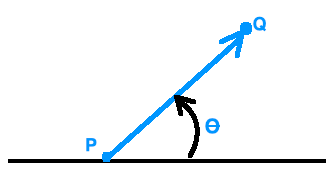Vector Notation

Vectors are denoted by lowercase letters or by their initial and terminal point.

In handwritten documents, a half arrow is placed over either notation. For example, in the picture above we could call the vector.

In printed documents, vector names are shown in bold print without the half arrow.

Standard Position of a Vector

A vector with its initial point at the origin of a Rectangular Coordinate System is said to be in standard position.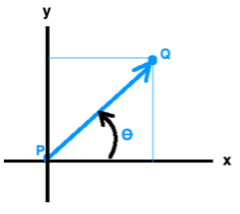Definition of the Component Form of a Vector

Given a vector in standard position, the coordinates of its terminal point, say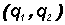, determine its component form, written as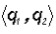where q1 and q2 are called components.

The component form of a vector requires angle brackets.

Finding the Component Form of a Vector - see #1 and 2 in the "Examples" document

If a vector is not in standard position, we can still find its component form. This also moves it to standard position.

Given a vector with initial point P atand terminal point Q at, its component form is found by.  As a memory aid remember "terminal minus initial" for the x- and y-coordinate!

Magnitude of a Vector - see #3 through 6 in the "Examples" document

This is the "length" of the vector.  It can also represent speed, weight, etc. The magnitude of a vector v =is denoted by ||v||.

It is found by using the Pythagorean Theorem on a vector in standard position and is

||v|| =.

NOTE: YOU MUST MEMORIZE THIS FORMULA!

Direction of a Vector - see #7 and 8 in the "Examples" document

Place the initial point of a vector at the origin in a coordinate system. Then the direction of the vector is given by the positive anglebetween the positive x-axis and the vector.We use the fact that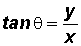which we derived in the lesson on polar coordinates using the following picture:Zero Vector

A zero vector can be denoted with a boldfaced 0 or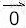. In component form it can be written as v = < 0, 0 >.

Vector Addition - see #9 in the "Examples" document

Let u =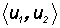and v =then u + v =.

The vector u + v is called the resultant vector.

Scalar Multiplication of Vectors - see #10 in the "Examples" document

In vector algebra, any real number is called scalar.

Let u =and let c be a scalar (a real number), then.

The Negative of a Vector - see #10 in the "Examples" document

Given vector v, its negative is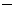v. It has the same magnitude as vector v, but points exactly in the opposite direction.

Vector Subtraction - see #10 in the "Examples" document

Vector subtraction is viewed as an addition of the negative of a vector.

uv = u + (v) = u1v